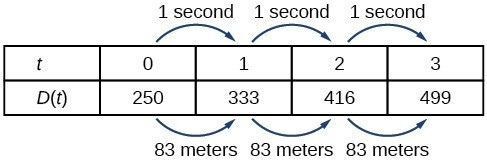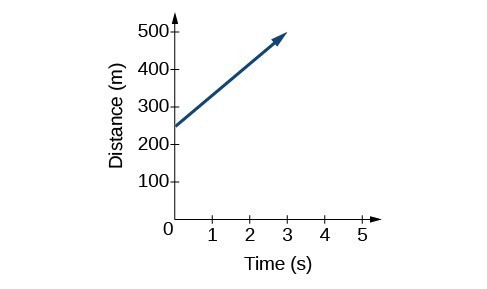## Represent a linear function

The function describing the train’s motion is a linear function, which is defined as a function with a constant rate of change, that is, a polynomial of degree 1. There are several ways to represent a linear function, including word form, function notation, tabular form, and graphical form. We will describe the train’s motion as a function using each method.

## Representing a Linear Function in Word Form

Let’s begin by describing the linear function in words. For the train problem we just considered, the following word sentence may be used to describe the function relationship.

• The train’s distance from the station is a function of the time during which the train moves at a constant speed plus its original distance from the station when it began moving at constant speed.

The speed is the rate of change. Recall that a rate of change is a measure of how quickly the dependent variable changes with respect to the independent variable. The rate of change for this example is constant, which means that it is the same for each input value. As the time (input) increases by 1 second, the corresponding distance (output) increases by 83 meters. The train began moving at this constant speed at a distance of 250 meters from the station.

## Representing a Linear Function in Function Notation

Another approach to representing linear functions is by using function notation. One example of function notation is an equation written in the form known as the slope-intercept form of a line, where $x$ is the input value, $m$ is the rate of change, and $b$ is the initial value of the dependent variable.

$\begin{cases}\text{Equation form}\hfill & y=mx+b\hfill \\ \text{Equation notation}\hfill & f\left(x\right)=mx+b\hfill \end{cases}$

In the example of the train, we might use the notation $D\left(t\right)$ in which the total distance $D$
is a function of the time $t$. The rate, $m$, is 83 meters per second. The initial value of the dependent variable $b$ is the original distance from the station, 250 meters. We can write a generalized equation to represent the motion of the train.

$D\left(t\right)=83t+250$

## Representing a Linear Function in Tabular Form

A third method of representing a linear function is through the use of a table. The relationship between the distance from the station and the time is represented in the table in Figure 1. From the table, we can see that the distance changes by 83 meters for every 1 second increase in time.Figure 1. Tabular representation of the function D showing selected input and output values

### Q & A

Can the input in the previous example be any real number?

No. The input represents time, so while nonnegative rational and irrational numbers are possible, negative real numbers are not possible for this example. The input consists of non-negative real numbers.

## Representing a Linear Function in Graphical Form

Another way to represent linear functions is visually, using a graph. We can use the function relationship from above, $D\left(t\right)=83t+250$, to draw a graph, represented in the graph in Figure 2. Notice the graph is a line. When we plot a linear function, the graph is always a line.

The rate of change, which is constant, determines the slant, or slope of the line. The point at which the input value is zero is the vertical intercept, or y-intercept, of the line. We can see from the graph that the y-intercept in the train example we just saw is $\left(0,250\right)$ and represents the distance of the train from the station when it began moving at a constant speed.Figure 2. The graph of $D\left(t\right)=83t+250$. Graphs of linear functions are lines because the rate of change is constant.

Notice that the graph of the train example is restricted, but this is not always the case. Consider the graph of the line $f\left(x\right)=2{x}_{}+1$. Ask yourself what numbers can be input to the function, that is, what is the domain of the function? The domain is comprised of all real numbers because any number may be doubled, and then have one added to the product.

### A General Note: Linear Function

A linear function is a function whose graph is a line. Linear functions can be written in the slope-intercept form of a line

$f\left(x\right)=mx+b$

where $b$ is the initial or starting value of the function (when input, $x=0$), and $m$ is the constant rate of change, or slope of the function. The y-intercept is at $\left(0,b\right)$.

### Example 1: Using a Linear Function to Find the Pressure on a Diver

The pressure, $P$, in pounds per square inch (PSI) on the diver in Figure 3 depends upon her depth below the water surface, $d$, in feet. This relationship may be modeled by the equation, $P\left(d\right)=0.434d+14.696$. Restate this function in words.Figure 3. (credit: Ilse Reijs and Jan-Noud Hutten)

### Solution

To restate the function in words, we need to describe each part of the equation. The pressure as a function of depth equals four hundred thirty-four thousandths times depth plus fourteen and six hundred ninety-six thousandths.

### Analysis of the Solution

The initial value, 14.696, is the pressure in PSI on the diver at a depth of 0 feet, which is the surface of the water. The rate of change, or slope, is 0.434 PSI per foot. This tells us that the pressure on the diver increases 0.434 PSI for each foot her depth increases.